### Home > CALC > Chapter 7 > Lesson 7.3.4 > Problem7-151

7-151.
1. Multiple Choice: If y = x + sin(xy), then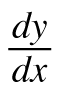=
Homework Help ✎

1. 1 + cos(xy)

2. 1 + y cos(xy​)

3.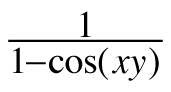4.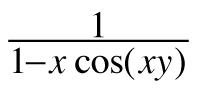5.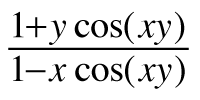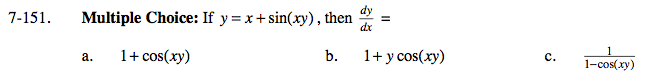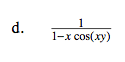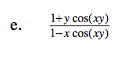Implicit differentiation with the Chain Rule can be messy.

$y'=1+\text{cos}(xy)\left ( y\frac{dx}{dx}+x\frac{dy}{dx} \right )$